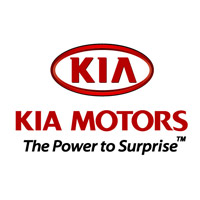# 起亚K3S本地参考价： 10.18 万元起 厂商指导价：10.18-14.38 万
 车市行情　｜　起亚K3S 降万
 最新年款： 2014款 级　别：紧凑型车 结　构：两厢车 车身颜色： 红色 油　耗：6.8L/100km 排　量：1.6 内饰配色： 红黑 保　修：3年或6万公里 变速箱：AT|MT

#### 东风悦达起亚KX3量产版于2015年3月上市#### 6款车获5星评价 2014第三批C-NCAP成绩#### 东风悦达起亚K3S购车手册 推荐GLS车型1.6L 自然吸气 128马力 国IV(京V) 官方指导价 本地参考价 车型信息 2014款 1.6L 手动GL 6挡手动 前置前驱 10.18万暂无 参数　图片　＋对比 2014款 1.6L 手动GLS 6挡手动 前置前驱 11.48万暂无 参数　图片　＋对比 2014款 1.6L 自动GLS 6挡手自一体 前置前驱 12.48万暂无 参数　图片　＋对比 2014款 1.6L 自动DLX 6挡手自一体 前置前驱 13.18万暂无 参数　图片　＋对比 2014款 1.6L 自动Premium 6挡手自一体 前置前驱 14.38万暂无 参数　图片　＋对比 2014款 1.6L 自动GL 6挡手自一体 前置前驱 11.18万暂无 参数　图片　＋对比

### 绕车实拍 外观 中控 座椅 细节 全部### 起亚K3S 新闻资讯#### 东风悦达起亚KX3量产版于2015年3月上市#### 6款车获5星评价 2014第三批C-NCAP成绩

2014年9月6日，2014年度C-NCAP第三批车型碰撞评价试验成绩正式公布，此次参加碰撞试验的共有8款车型，其中包括丰田86、起亚K3S、日产新奇骏、...#### 东风悦达起亚K3S购车手册 推荐GLS车型#### 东风悦达起亚K3S浏阳区域上市

2014年4月12日备受瞩目的东风悦达起亚K3S于浏阳体育中心型动上市。K3S不仅传承了DesignKia设计语言赋予K系列车型的动感基因，更融汇新锐的灵动...#### 起亚K3S借力大河车展“型”动上市#### 两厢更好看 实拍顶配东风悦达起亚K3S#### 驾驶感受运动化 试驾东风悦达起亚K3S

K3S是东风悦达起亚推出的最新车型，基于美版Forte两厢版改进而来，所以无论从外观上还是车系名字，都给人感觉这不就是两厢版的K3吗？说实话在...#### K3S团购活动圆满结束#### “时尚不止 潮流不息”诚盛起亚K3S魅力绽放

K3S是东风悦达起亚打造的“型车”概念完美诠释。K3S创新设计的狭长前中网与大面积梯形进气口搭配，充满视觉张力而又不失个性。犀利的氙气大灯...#### 中秋之夜邂逅 男神之车K3S#### 诚盛起亚助首届大型试驾会#### 诚盛起亚店邀您一起 名“诚”钜惠

5月10日-5月11日，第七届名城汽车巡展在新乡市体育中心拉开帷幕。作为本次东风悦达起亚新乡站的参展商新乡诚盛4S店，特在此次活动中将推出劲爆...#### 东风悦达起亚K3S联袂李敏镐型动上市

2014年4月12日，备受瞩目的东风悦达起亚K3S在郑州地区型动上市，东风悦达起亚厂家代表、郑州地区经销商代表及省内各主流媒体参加了上市发布会...#### K3S“型”动新车上市#### 心“零”相惜 “零”元预订K3S### 猜你喜欢

﻿
• 快速找车
• 选择品牌
• 选择品牌
• A  奥迪
• A  阿斯顿·马丁
• A  阿尔法·罗密欧
• B  宝沃
• B  布加迪
• B  巴博斯
• B  保时捷
• B  宾利
• B  奔驰
• B  宝马
• B  本田
• B  别克
• B  标致
• B  比亚迪
• B  宝骏
• B  北汽制造
• B  北汽新能源
• B  北汽幻速
• B  北汽威旺
• B  北京汽车
• B  奔腾
• B  北汽绅宝
• B  北汽昌河
• C  长安欧尚
• C  长安
• C  长安凯程
• C  长城
• D  大众
• D  道奇
• D  DS
• D  东南
• D  东风风神
• D  东风风行
• D  东风小康
• D  东风风度
• D  东风
• F  福特
• F  丰田
• F  菲亚特
• F  法拉利
• F  福田
• F  福迪
• F  福汽启腾
• G  观致
• G  广汽传祺
• G  广汽吉奥
• G  GMC
• H  红旗
• H  汉腾汽车
• H  哈弗
• H  哈飞
• H  海格
• H  海马
• H  华颂
• H  黄海
• H  华泰
• H  恒天
• J  捷途
• J  几何汽车
• J  捷达
• J  吉利汽车
• J  捷豹
• J  Jeep
• J  江淮
• J  江铃
• J  金杯
• J  九龙
• J  金旅
• K  凯翼
• K  凯迪拉克
• K  克莱斯勒
• K  科尼塞克
• K  卡威
• K  开瑞
• L  路虎
• L  林肯
• L  劳斯莱斯
• L  兰博基尼
• L  雷克萨斯
• L  铃木
• L  领克
• L  雷诺
• L  理念
• L  力帆
• L  莲花汽车
• L  猎豹
• L  路特斯
• L  陆风
• M  马自达
• M  MG
• M  MINI
• M  玛莎拉蒂
• M  摩根
• M  迈凯轮
• N  纳智捷
• O  欧拉
• O  欧宝
• O  讴歌
• O  欧朗
• Q  奇瑞
• Q  起亚
• Q  启辰
• R  日产
• R  荣威
• R  瑞麒
• S  SERES赛力斯
• S  三菱
• S  斯威汽车
• S  萨博
• S  smart
• S  斯柯达
• S  斯巴鲁
• S  思铭
• S  双龙
• S  上汽大通
• S  双环
• T  特斯拉
• T  腾势
• W  蔚来
• W  沃尔沃
• W  WEY
• W  五菱汽车
• W  五十铃
• W  威兹曼
• W  威麟
• X  现代
• X  雪佛兰
• X  星途
• X  雪铁龙
• X  小鹏汽车
• X  西雅特
• Y  一汽
• Y  英菲尼迪
• Y  英致
• Y  依维柯
• Y  野马汽车
• Y  永源
• Z  众泰
• Z  中华
• Z  中兴
• Z  知豆
• 选择车系
• 选择车系
• 车型对比
• 选择品牌
• 选择品牌
• A  奥迪
• A  阿斯顿·马丁
• A  阿尔法·罗密欧
• B  宝沃
• B  布加迪
• B  巴博斯
• B  保时捷
• B  宾利
• B  奔驰
• B  宝马
• B  本田
• B  别克
• B  标致
• B  比亚迪
• B  宝骏
• B  北汽制造
• B  北汽新能源
• B  北汽幻速
• B  北汽威旺
• B  北京汽车
• B  奔腾
• B  北汽绅宝
• B  北汽昌河
• C  长安欧尚
• C  长安
• C  长安凯程
• C  长城
• D  大众
• D  道奇
• D  DS
• D  东南
• D  东风风神
• D  东风风行
• D  东风小康
• D  东风风度
• D  东风
• F  福特
• F  丰田
• F  菲亚特
• F  法拉利
• F  福田
• F  福迪
• F  福汽启腾
• G  观致
• G  广汽传祺
• G  广汽吉奥
• G  GMC
• H  红旗
• H  汉腾汽车
• H  哈弗
• H  哈飞
• H  海格
• H  海马
• H  华颂
• H  黄海
• H  华泰
• H  恒天
• J  捷途
• J  几何汽车
• J  捷达
• J  吉利汽车
• J  捷豹
• J  Jeep
• J  江淮
• J  江铃
• J  金杯
• J  九龙
• J  金旅
• K  凯翼
• K  凯迪拉克
• K  克莱斯勒
• K  科尼塞克
• K  卡威
• K  开瑞
• L  路虎
• L  林肯
• L  劳斯莱斯
• L  兰博基尼
• L  雷克萨斯
• L  铃木
• L  领克
• L  雷诺
• L  理念
• L  力帆
• L  莲花汽车
• L  猎豹
• L  路特斯
• L  陆风
• M  马自达
• M  MG
• M  MINI
• M  玛莎拉蒂
• M  摩根
• M  迈凯轮
• N  纳智捷
• O  欧拉
• O  欧宝
• O  讴歌
• O  欧朗
• Q  奇瑞
• Q  起亚
• Q  启辰
• R  日产
• R  荣威
• R  瑞麒
• S  SERES赛力斯
• S  三菱
• S  斯威汽车
• S  萨博
• S  smart
• S  斯柯达
• S  斯巴鲁
• S  思铭
• S  双龙
• S  上汽大通
• S  双环
• T  特斯拉
• T  腾势
• W  蔚来
• W  沃尔沃
• W  WEY
• W  五菱汽车
• W  五十铃
• W  威兹曼
• W  威麟
• X  现代
• X  雪佛兰
• X  星途
• X  雪铁龙
• X  小鹏汽车
• X  西雅特
• Y  一汽
• Y  英菲尼迪
• Y  英致
• Y  依维柯
• Y  野马汽车
• Y  永源
• Z  众泰
• Z  中华
• Z  中兴
• Z  知豆
• 选择车系
• 选择车系
• 选择车型
• 选择车型
• 意见反馈